# Difference between revisions of "Poincaré equations"

General equations of the mechanics of holonomic systems (cf. Holonomic system) presented with the help of a certain Lie algebra of infinitesimal transformations.

Let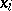,, be variables determining the position of a holonomic mechanical system constrained by ideal constraints depending explicitly on time. If the system hasdegrees of freedom, then there is an intransitive algebra of infinitesimal transformations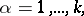enabling the system to be carried at the moment of timefrom the positionto the infinitesimally-near actual positionand the virtual positionby infinitesimal transformationsof the algebra andof the subalgebra, respectively. Here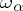and, the independent variables determining the virtual and actual displacements respectively, are connected by the equations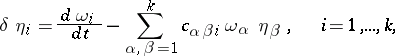if the algebra of virtual displacements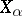is determined by its structure constants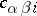: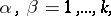and the operator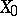commutes with the algebra of virtual displacements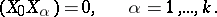Below these conditions are assumed to hold.

The Poincaré equations are ordinary first-order (with respect to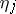) differential equations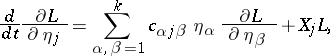(1)

where,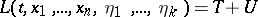is the Lagrange function,is the kinetic energy andis the force function.

The equations (1) were first obtained by H. Poincaré (see ) in the case when the algebra of virtual displacements is transitive and the constraints do not depend explicitly on time, and he applied them (see ) to investigate the motion of a solid body with an ellipsoidal cavity, entirely filled by a uniformly vortex moving ideal fluid. N.G. Chetaev (see ) generalized and developed the theory of the Poincaré equations to the case where the algebra of displacements is intransitive and the constraints depend explicitly on time (see ) and also converted them to a simpler canonical form (see Chetaev equations). In particular, he gave (see ) a method for constructing the algebra of virtual and actual displacements when the holonomic constraints are given by a differential form and he introduced the important concept of cyclic displacements.

Displacements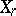,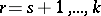, are said to be cyclic if they satisfy the conditions: 1)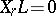; 2)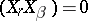,,.

According to 2) the cyclic displacementsform an Abelian subalgebra of the algebra of virtual displacements which commutes with all the operators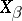. For cyclic displacements the first integrals of the Poincaré equations,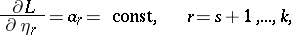exist. From these relations the variables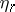can be expressed in terms of the constants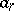and the variables,,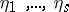, and one can introduce the Routh function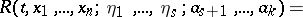Then for the non-cyclic displacements the Poincaré equations take the form(2)After integrating the equations (2) the valuesare determined by the equations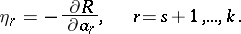If, in addition,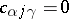,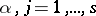,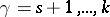, that is, if the non-cyclic displacements,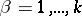, form a subalgebra of the algebra of virtual displacements, then the mechanical system corresponding to this subalgebra forms some sort of independent holonomic system withdegrees of freedom, described by the equations (1) when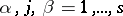, where the role of the functionis played by the function.

The Poincaré equations contain as particular cases: the Lagrange equations (in mechanics) when the transformation algebra of infinitesimal increments in one of the variables reduces to a commutative algebra of transformations; and the Euler equation for the rotation of a solid body, when theare taken to be the projections,andof the instantaneous angular velocity.